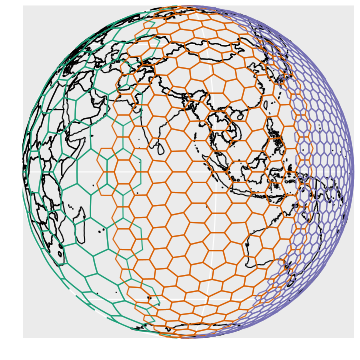#Spatial Analysis Done Right

You want to do spatial statistics, and it's going to involve binning.

Binning with a rectangular grid introduces messy distortions. At the macro-scale using a rectangular grid does things like making Greenland bigger than the United States and Antarctica the largest continent.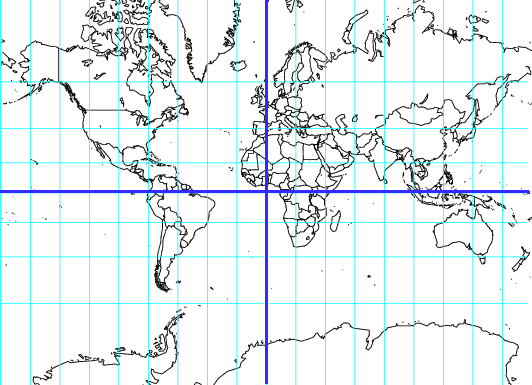But this kind of distortion is present no matter what the resolution is.

What you want are bins of equal size, regardless of where they are on the globe, regardless of their resolution.

dggridR solves this problem.

dggridR builds discrete global grids which partition the surface of the Earth into hexagonal, triangular, or diamond cells, all of which have the same size. (There are some minor caveats which are detailed in the vignettes.)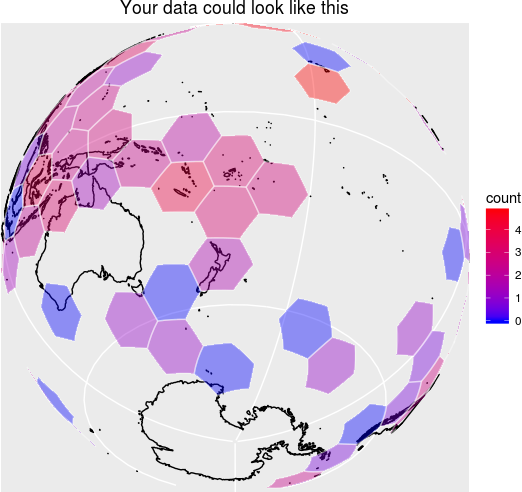This package includes everything you need to make spatial binning great again.

Many details are included in the vignette.

#Grids

The following grids are available:

• ISEA3H: Icosahedral Snyder Equal Area Aperture 3 Hexagonal Grid
• ISEA4H: Icosahedral Snyder Equal Area Aperture 4 Hexagonal Grid
• ISEA43H: Icosahedral Snyder Equal Area Mixed Aperture 4,3 Hexagonal Grid
• ISEA4T: Icosahedral Snyder Equal Area Aperture 4 Triangular Grid
• ISEA4D: Icosahedral Snyder Equal Area Aperture 4 Diamond Grid
• FULLER3H: Fuller Aperature 3 Hexagonal Grid
• FULLER4H: Fuller Aperature 4 Hexagonal Grid
• FULLER43H: Fuller Mixed Aperature 4,3 Hexagonal Grid
• FULLER4T: Fuller Aperature 4 Triganular Grid
• FULLER4D: Fuller Aperature 4 Diamond Grid

Unless you are using cells with very large areas (significant fractions of Earth's hemispheres), I recommend the ISEA3H be your default grid.

This grid, along with the other Icosahedral grids ensures that all cells are of equal area, with a notable exception. At every resolution, the Icosahedral grids contain 12 pentagonal cells which each have an area exactly 5/6 that of the hexagonal cells. But you don't need to worry about this too much for two reasons. (1) As the table below shows, these cells are a small, small minority of the total number of cells. (2) The grids are orientated so that these cells are in out-of-the-way places. Future versions of this package will allow you to reorient the grids, if need be. (TODO)

For more complex applications than simple spatial binning, it is necessary to consider trade-offs between the different grids. Good references for understanding these include [@Kimerling1999; @Gregory2008].

Users attempting multi-scale analyses should be aware that in the hexagonal grids cells from one resolution level are partially contained by the cells of other levels.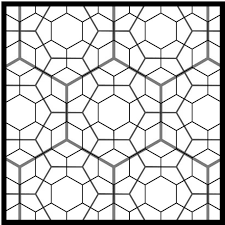At present, there is no convenient way to convert grid cell ids at one resolution level to another. In the future, I hope to add this capability to the package. (TODO)

##ISEA3H Details

The following chart shows the number of cells, their area, and statistics regarding the spacing of their center nodes for the ISEA3H grid type.

Res Number of Cells Cell Area (km2) Min Max Mean Std
0 12 51,006,562.17241
1 32 17,002,187.39080 4,156.18000 4,649.10000 4,320.49000 233.01400
2 92 5,667,395.79693 2,324.81000 2,692.72000 2,539.69000 139.33400
3 272 1,889,131.93231 1,363.56000 1,652.27000 1,480.02000 89.39030
4 812 629,710.64410 756.96100 914.27200 855.41900 52.14810
5 2,432 209,903.54803 453.74800 559.23900 494.95900 29.81910
6 7,292 69,967.84934 248.80400 310.69300 285.65200 17.84470
7 21,872 23,322.61645 151.22100 187.55000 165.05800 9.98178
8 65,612 7,774.20548 82.31100 104.47000 95.26360 6.00035
9 196,832 2,591.40183 50.40600 63.00970 55.02260 3.33072
10 590,492 863.80061 27.33230 35.01970 31.75960 2.00618
11 1,771,472 287.93354 16.80190 21.09020 18.34100 1.11045
12 5,314,412 95.97785 9.09368 11.70610 10.58710 0.66942
13 15,943,232 31.99262 5.60065 7.04462 6.11367 0.37016
14 47,829,692 10.66421 3.02847 3.90742 3.52911 0.22322
15 143,489,072 3.55473 1.86688 2.35058 2.03789 0.12339
16 430,467,212 1.18491 1.00904 1.30335 1.17638 0.07442
17 1,291,401,632 0.39497 0.62229 0.78391 0.67930 0.04113
18 3,874,204,892 0.13166 0.33628 0.43459 0.39213 0.02481
19 11,622,614,672 0.04389 0.20743 0.26137 0.22643 0.01371
20 34,867,844,012 0.01463 0.11208 0.14489 0.13071 0.00827

#How do I use it?

1. Construct a discrete global grid system (dggs) object using `dgconstruct()`

2. Get information about your dggs object using:

``````* `dggetres()`
* `dginfo()`
* `dgmaxcell()`
``````
1. Get the grid cells of some lat-long points with:
``````* `dgGEO_to_SEQNUM()`
* One of many, many other such functions
``````
1. Get the boundaries of the associated grid cells for use in plotting with:
``````* `dgcellstogrid()`
* `dgearthgrid()`
* `dgrectgrid()`
* `dgshptogrid()`
``````
1. Check that your dggs object is valid (if you've mucked with it) using:
``````* `dgverify()`
``````

#Examples

##Binning Lat-Long Points

The following example demonstrates converting lat-long locations (the epicenters of earthquakes) to discrete global grid locations (cell numbers), binning based on these numbers, and plotting the result. Additionally, the example demonstrates how to get the center coordinates of the cells.

``````#Include libraries
library(dggridR)
library(dplyr)

#Construct a global grid with cells approximately 1000 miles across
dggs          <- dgconstruct(spacing=1000, metric=FALSE, resround='down')

#Load included test data set
data(dgquakes)

#Get the corresponding grid cells for each earthquake epicenter (lat-long pair)
dgquakes\$cell <- dgGEO_to_SEQNUM(dggs,dgquakes\$lon,dgquakes\$lat)\$seqnum

#Converting SEQNUM to GEO gives the center coordinates of the cells
cellcenters   <- dgSEQNUM_to_GEO(dggs,dgquakes\$cell)

#Get the number of earthquakes in each cell
quakecounts   <- dgquakes %>% group_by(cell) %>% summarise(count=n())

#Get the grid cell boundaries for cells which had quakes
grid          <- dgcellstogrid(dggs,quakecounts\$cell,frame=TRUE,wrapcells=TRUE)

#Update the grid cells' properties to include the number of earthquakes
#in each cell
grid          <- merge(grid,quakecounts,by.x="cell",by.y="cell")

#Make adjustments so the output is more visually interesting
grid\$count    <- log(grid\$count)
cutoff        <- quantile(grid\$count,0.9)
grid          <- grid %>% mutate(count=ifelse(count>cutoff,cutoff,count))

#Get polygons for each country of the world
countries <- map_data("world")
``````

Okay, let's draw the plot. Notice how the hexagons appear to be all different sizes. Really, though, they're not: that's just the effect of trying to plot a sphere on a flat surface! And that's what would happen to your data if you didn't use this package :-)

``````#Plot everything on a flat map
p<- ggplot() +
geom_polygon(data=countries, aes(x=long, y=lat, group=group), fill=NA, color="black")   +
geom_polygon(data=grid,      aes(x=long, y=lat, group=group, fill=count), alpha=0.4)    +
geom_path   (data=grid,      aes(x=long, y=lat, group=group), alpha=0.4, color="white") +
geom_point  (aes(x=cellcenters\$lon_deg, y=cellcenters\$lat_deg)) +
p
``````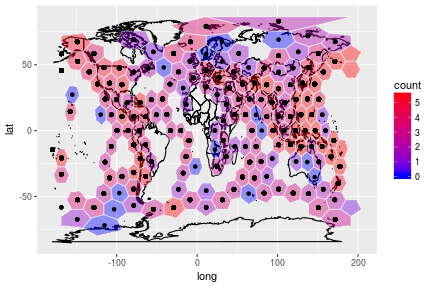If we replot things on a sphere, it's easy to see that all of the hexagons are the same size, as they should be. Note how they deal easily with the longitudinal convergence towards Antarctica, as well as with crossing -180/180 degrees.

``````#Replot on a spherical projection
p+coord_map("ortho", orientation = c(-38.49831, -179.9223, 0))+
xlab('')+ylab('')+
theme(axis.ticks.x=element_blank())+
theme(axis.ticks.y=element_blank())+
theme(axis.text.x=element_blank())+
theme(axis.text.y=element_blank())+
ggtitle('Your data could look like this')
``````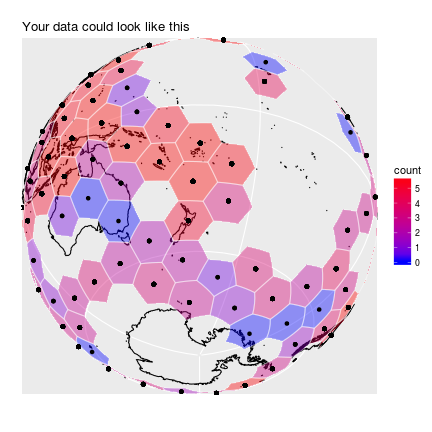You can also write out a KML file with your data included for displaying in, say, Google Earth:

``````library(rgdal)

#Get the grid cell boundaries for the whole Earth using this dggs in a form
#suitable for printing to a KML file
grid          <- dgearthgrid(dggs,frame=FALSE)

#Update the grid cells' properties to include the number of earthquakes
#in each cell
grid@data\$count <- merge(grid@data, quakecounts, by.x="cell", by.y="cell", all.x=TRUE)\$count

#Write out the grid
writeOGR(grid, "quakes_per_cell.kml", "quakes", "KML")
``````

##Randomly Sampling the Earth: Method 1

Say you want to sample `N` areas of equal size uniformly distributed on the Earth. dggridR provides two possible ways to accomplish this. The conceptually simplest is to choose `N` uniformly distributed lat-long pairs and retrieve their associated grid cells:

``````#Include libraries
library(dggridR)
library(rgdal)
library(dplyr)

N <- 100    #How many cells to sample

#Distribute the points uniformly on a sphere using equations from
#http://mathworld.wolfram.com/SpherePointPicking.html
u     <- runif(N)
v     <- runif(N)
theta <- 2*pi*u      * 180/pi
phi   <- acos(2*v-1) * 180/pi
lon   <- theta-180
lat   <- phi-90

df    <- data.frame(lat=lat,lon=lon)

#Construct a global grid in which every hexagonal cell has an area of
#100,000 miles^2. You could, of course, choose a much smaller value, but these
#will show up when I map them later.

#Note: Cells can only have certain areas, the `dgconstruct()` function below
#will tell you which area is closest to the one you want. You can also round
#up or down.

#Note: 12 cells are actually pentagons with an area 5/6 that of the hexagons
#But, with millions and millions of hexes, you are unlikely to choose one
#Future versions of the package will make it easier to reject the pentagons
dggs    <- dgconstruct(area=100000, metric=FALSE, resround='nearest')

#Get the corresponding grid cells for each randomly chosen lat-long
df\$cell <- dgGEO_to_SEQNUM(dggs,df\$lon,df\$lat)\$seqnum

#Get the hexes for each of these cells
gridfilename <- dgcellstogrid(dggs,df\$cell)
``````

The resulting distribution of cells appears as follows:

``````#Get the grid in a more convenient format
grid           <- dgcellstogrid(dggs,df\$cell,frame=TRUE,wrapcells=TRUE)

#Get polygons for each country of the world
countries      <- map_data("world")

#Plot everything on a flat map
p<- ggplot() +
geom_polygon(data=countries, aes(x=long, y=lat, group=group), fill=NA, color="black")   +
geom_polygon(data=grid,      aes(x=long, y=lat, group=group), fill="green", alpha=0.4)    +
geom_path   (data=grid,      aes(x=long, y=lat, group=group), alpha=0.4, color="white")
p
``````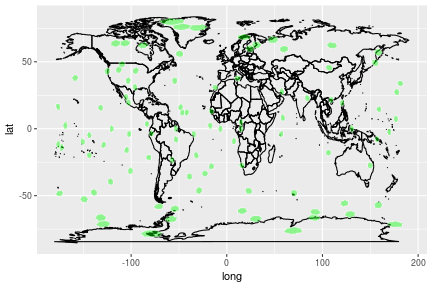##Randomly Sampling the Earth: Method 2

Say you want to sample `N` areas of equal size uniformly distributed on the Earth. dggridR provides two possible ways to accomplish this. The easiest way to do this is to note that grid cells are labeled from 1 to `M`, where `M` is the largest cell id at the resolution in question. Therefore, we can sample cell ids and generate a grid accordingly.

``````#Include libraries
library(dggridR)
library(dplyr)

N <- 100    #How many cells to sample

#Construct a global grid in which every hexagonal cell has an area of
#100,000 miles^2. You could, of course, choose a much smaller value, but these
#will show up when I map them later.

#Note: Cells can only have certain areas, the `dgconstruct()` function below
#will tell you which area is closest to the one you want. You can also round
#up or down.

#Note: 12 cells are actually pentagons with an area 5/6 that of the hexagons
#But, with millions and millions of hexes, you are unlikely to choose one
#Future versions of the package will make it easier to reject the pentagons
dggs    <- dgconstruct(area=100000, metric=FALSE, resround='nearest')

maxcell <- dgmaxcell(dggs)                     #Get maximum cell id
cells   <- sample(1:maxcell, N, replace=FALSE) #Choose random cells
grid    <- dgcellstogrid(dggs,cells,frame=TRUE,wrapcells=TRUE) #Get grid
``````

The resulting distribution of cells appears as follows:

``````#Get the grid in a more convenient format
grid           <- dgcellstogrid(dggs,df\$cell,frame=TRUE,wrapcells=TRUE)

#Get polygons for each country of the world
countries      <- map_data("world")

#Plot everything on a flat map
p<- ggplot() +
geom_polygon(data=countries, aes(x=long, y=lat, group=group), fill=NA, color="black")   +
geom_polygon(data=grid,      aes(x=long, y=lat, group=group), fill="green", alpha=0.4)    +
geom_path   (data=grid,      aes(x=long, y=lat, group=group), alpha=0.4, color="white")
p
``````##Save a grid for use in other software

Sometimes you want to use a grid in software other than R. To faciliate this, the grid generation commands include the `savegrid` argument, as demonstrated below.

``````library(dggridR)
#Generate a global grid whose cells are ~100,000 miles^2
dggs         <- dgconstruct(area=100000, metric=FALSE, resround='nearest')
#Save the cells to a KML file for use in other software
gridfilename <- dgearthgrid(dggs,savegrid=tempfile())
``````

##Get a grid that covers South Africa

``````library(dggridR)

#Generate a dggs specifying an intercell spacing of ~25 miles
dggs      <- dgconstruct(spacing=100, metric=FALSE, resround='nearest')

#Read in the South Africa's borders from the shapefile

#Get a grid covering South Africa
sa_grid   <- dgshptogrid(dggs, dg_shpfname_south_africa())

#Plot South Africa's borders and the associated grid
p<- ggplot() +
geom_polygon(data=sa_border, aes(x=long, y=lat, group=group), fill=NA, color="black")   +
geom_polygon(data=sa_grid,   aes(x=long, y=lat, group=group), fill="blue", alpha=0.4)   +
geom_path   (data=sa_grid,   aes(x=long, y=lat, group=group), alpha=0.4, color="white") +
coord_equal()
p
``````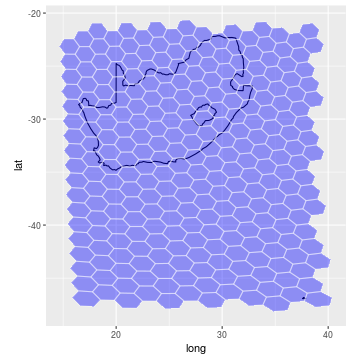#Caveats

At every resolution, the Icosahedral grids contain 12 pentagonal cells which each have an area exactly 5/6 that of the hexagonal cells. In the standard orientation, these are located as follows (scaled to a size corresponding to the grid resolution):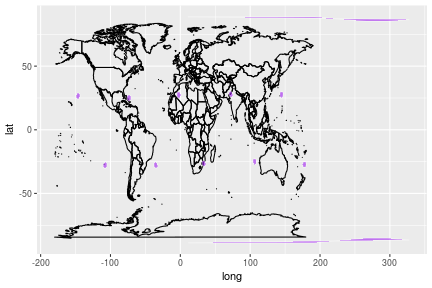• Method to convert between grid cell ids at different resolutions

• In the future, I plan to switch the package from using Kevin Sahr's dggrid software to the discrete global grid system standards currently being developed by OpenGeospatial. Those standards are being developed by a software working group right now, but will one day be released. At that point, I expect that GDAL/OGR/PROJ4 will incorporate the new standards making wider interoperability possible. Until that time, Sahr's dggrid is the best option I've found.

#Credits

This R package was developed by Richard Barnes (http://rbarnes.org).

The dggrid conversion software was developed predominantly by Kevin Sahr (http://www.discreteglobalgrids.org), with contributions from a few others.

Large portions of the above documentation are drawn from the DGGRID version 6.2b User Documentation, which is available in its entirety here.

#Disclaimer

This package should operate in the manner described here, in the package's main documentation, and in Kevin Sahr's dggrid documentation. Unfortunately, none of us are paid enough to make absolutely, doggone certain that that's the case. Use at your own discretion. That said, if you find bugs or are seeking enhancements, we want to hear about them.

#Citing this Package

Please cite this package as:

Richard Barnes (2017). dggridR: Discrete Global Grids for R. R package version 0.1.12. https://github.com/r-barnes/dggridR/

#References